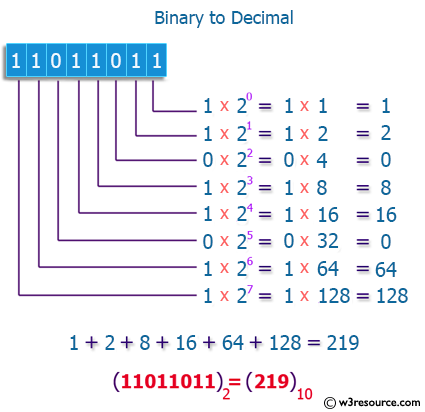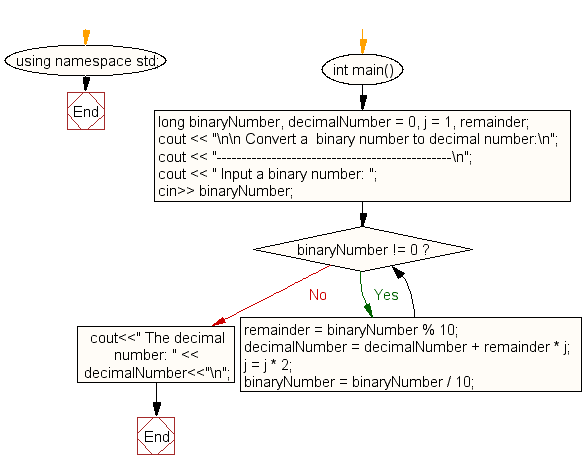﻿ C++ Exercises: Convert a binary number to decimal number - w3resource

# C++ Exercises: Convert a binary number to decimal number

## C++ For Loop: Exercise-73 with Solution

Write a C++ program to convert a binary number to a decimal number.

Pictorial Presentation:Sample Solution:-

C++ Code :

``````#include <iostream>
#include <math.h>
using namespace std;

int main()
{
long binaryNumber, decimalNumber = 0, j = 1, remainder;
cout << "\n\n Convert a  binary number to decimal number:\n";
cout << "-----------------------------------------------\n";
cout << " Input a binary number: ";
cin>> binaryNumber;
while (binaryNumber != 0)
{
remainder = binaryNumber % 10;
decimalNumber = decimalNumber + remainder * j;
j = j * 2;
binaryNumber = binaryNumber / 10;
}
cout<<" The decimal number: " << decimalNumber<<"\n";
}
``````

Sample Output:

```Convert a  binary number to decimal number:
-----------------------------------------------
Input a binary number: 1011
The decimal number: 11
```

Flowchart:C++ Code Editor: